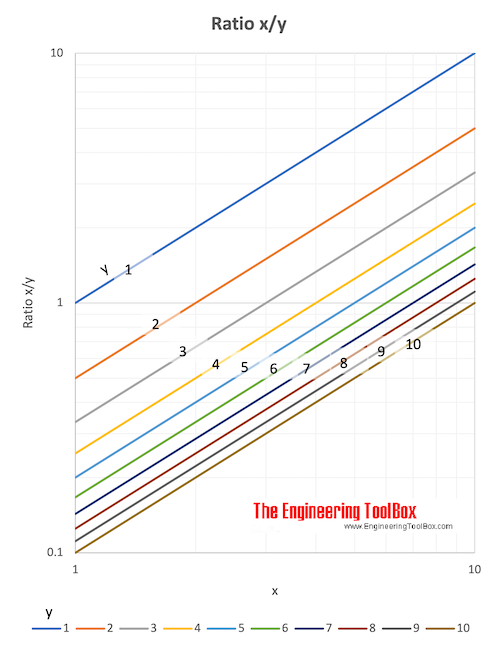Engineering ToolBox - Resources, Tools and Basic Information for Engineering and Design of Technical Applications!

# Ratios and Proportions

## The relative values between quantities - ratios and proportions.

### Ratio

The ratio of one quantity to an other is a fraction - and the number of times one quantity is contained in another quantity of the same kind.

The ratio between two quantities is the quotient obtained by dividing the first quantity by the second.

#### Example - Ratio

The ratio between 3 and 6 can be expressed as

3 / 6 = 1 / 2 = 0.5

Ratio can also be indicated by the sign ":" - like 3 : 6 or 1 : 2.### Reciprocal or Inverse Ratio

The reciprocal or inverse ratio is the opposite of the original ratio.

#### Example the Inverse Ratio

The inverse ratio between 6 and 3 can be expressed as

6 : 3  = 2 : 1 = 2

### Proportion

Proportion is the equality of ratios.

1 : 2 = 3 : 6

or

1 : 2 :: 3 : 6

## Related Topics

• ### Basics

The SI-system, unit converters, physical constants, drawing scales and more.
• ### Mathematics

Mathematical rules and laws - numbers, areas, volumes, exponents, trigonometric functions and more.

## Related Documents

• ### Energy Balance and Energy Ratio

Energy balance and energy payback ratio.
• ### Fractions

Law of fractions
• ### Movement Ratio

Machines and movement ratio (velocity ratio).
• ### Percentage

Working with relative values.
• ### Poisson's Ratio

When a material is stretched in one direction it tends to get thinner in the other two directions.
• ### Poisson's Ratios Metals

Some metals and their Poisson's Ratios.

## Engineering ToolBox - SketchUp Extension - Online 3D modeling!

Add standard and customized parametric components - like flange beams, lumbers, piping, stairs and more - to your Sketchup model with the Engineering ToolBox - SketchUp Extension - enabled for use with older versions of the amazing SketchUp Make and the newer "up to date" SketchUp Pro . Add the Engineering ToolBox extension to your SketchUp Make/Pro from the Extension Warehouse !

We don't collect information from our users. More about

## Citation

• The Engineering ToolBox (2007). Ratios and Proportions. [online] Available at: https://www.engineeringtoolbox.com/ratio-proportion-d_1300.html [Accessed Day Month Year].

Modify the access date according your visit.

10.2.10